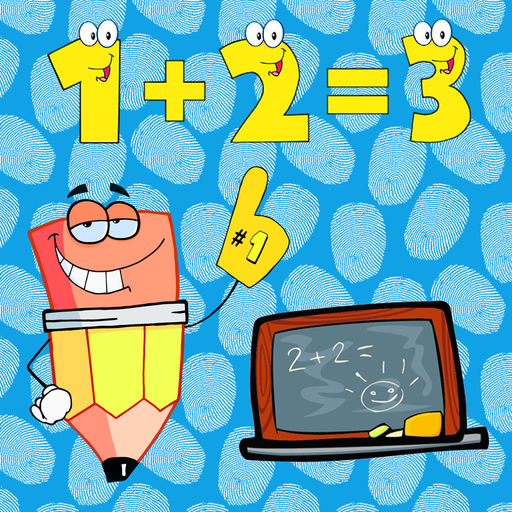Adding and Subtracting Equation - PreK Mathematical Formula# Adding and Subtracting Equation - PreK Mathematical Formula

## Suparerk Tatiyapornpun

Free
0

0 Ratings

Release Date

2016-03-17

Size

9.6 MB

### Screenshots for iPhone

iPhone
Adding and Subtracting Equation - PreK Mathematical Formula Description
The game for kids education used to learn how to do addition and subtraction with pictures and equation. The game application provides addition and subtraction from 1 to 100 in English user interface with funny graphics, animations, and sound effect.
Adding and Subtracting Equation - PreK Mathematical Formula 1.0 Update
2016-03-17 Version History
This update is signed with Apple’s latest signing certificate. No new features are included.
More